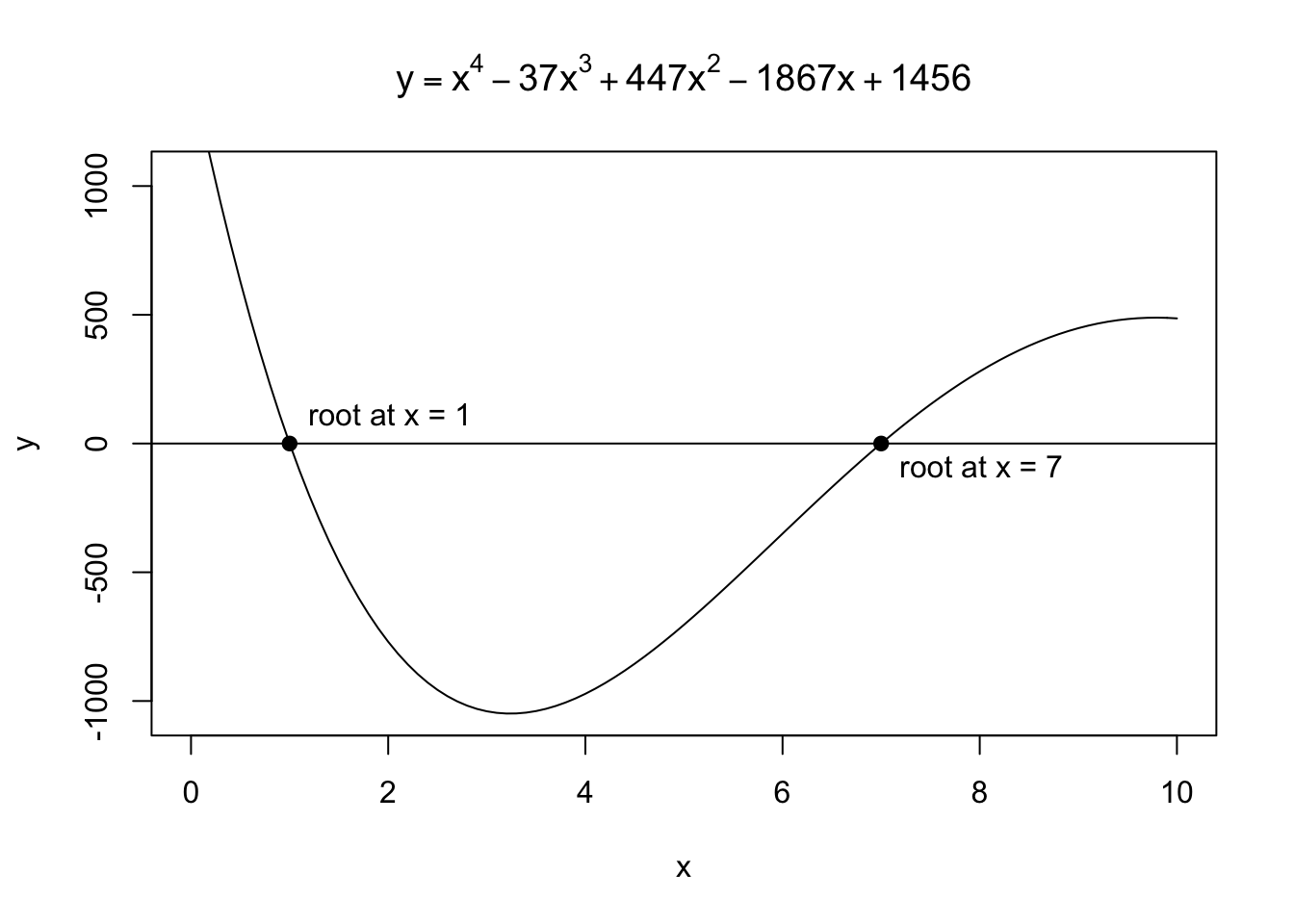These notes are based on Chapter 10 of Jones, O., Maillardet, R., & Robinson, A. (2009). Introduction to scientific programming and simulation using R. CRC Press.

# Introduction

A root is a solution of $$f(x)=0$$. Visually, a root is a point where the graph $$y=f(x)$$ crosses the x-axis. For example, in the interval $$[0,10]$$, there are two roots of the following $$f$$:

plot(c(1,7), c(0,0), pch = 19,
xlim=c(0,10), ylim=c(-1050,1050),
xlab="x", ylab="y",
main=expression(y==x^4-37*x^3+447*x^2-1867*x+1456))
abline(h=0)
text(1, 100, "root at x = 1", pos=4)
text(7, -100, "root at x = 7", pos=4)Root finding is important because:

• Factorisation of a polynomial, one of the most popular classes of functions (e.g., Taylor series approximation). For example, \begin{align} f(x) &= x^4-37x^3+447x^2-1867x+1456\\ &= (x-1)(x-7)(x-13)(x-16) \end{align}
• Many problems can be modelled as root finding of suitable functions. Remember that finding a root of $$f(x)$$ is equivalent to solving an equation $$g(x)=h(x)$$ where $$f(x) = g(x)-h(x)$$.

## Loan repayments

Let’s suppose:

• $$P_n$$ : Remaining amount of loan after $$n$$ months
• $$P_0$$ : Initial amount of loan
• $$N$$ : Duration of repayments
• $$A$$ : Monthly repayment amount
• $$r$$ : Monthly interest rate

Given $$P_{n-1}$$, we know: $P_n = (1+r)P_{n-1} - A$ That is, each month, interest based on $$P_{n-1}$$ is added and then you repay $$A$$. The solution of the difference equation (i.e., the expression of $$P_n$$ as a function of $$n$$) is:

$P_n = (1+r)^nP_0 - \frac{A}{r}\left((1+r)^n-1\right) .$

To see this, for each $$k\in\{1,\dots,n\}$$, multiply both sides of $$P_k = (1+r)P_{k-1} - A$$ by $$(1+r)^{n-k}$$ and get a telescoping sum.

We negotiate with a bank on the interest rate. A question is under what $$r$$, can we repay the loan after $$N$$ months?

We could get the answer by solving $$P_N=0$$ for $$r$$: \begin{alignat}{2} && P_N &= 0\\ &\Leftrightarrow \quad & (1+r)^NP &= \frac{A}{r}\left((1+r)^N-1\right)\\ &\Leftrightarrow \quad & (1+r)^N &= \frac{A}{A-rP}\\ &&&\vdots \end{alignat} Well, this is hard (at least for me). Instead, we may use numerical root-finding methods for $$f(r):=P_N$$.

# Fixed-point iteration

## Fixed point

Let $$g: \mathbb{R}\to\mathbb{R}$$ be a continuous function. Then, a fixed point of $$g$$ is a solution of $$g(x)=x$$. Visually, a fixed point is a point where the graph $$y=g(x)$$ crosses the 45-degree line. For example, a hyperbolic tangent $g(x) = 2\tanh(x) = 2\frac{e^{x}-e^{-x}}{e^{x}+e^{-x}}$ has three fixed points: $$x = -1.915, 0, 1.915$$.

x <- c(-1.915,0,1.915)
y <- c(-1.915,0,1.915)
plot(x, y, pch = 19, xlim=c(-2,2), ylim=c(-2,2))
title(expression(y==2*tanh(x)))
arrows(1.8, 1, 1.9, 1.8, length=0.1)